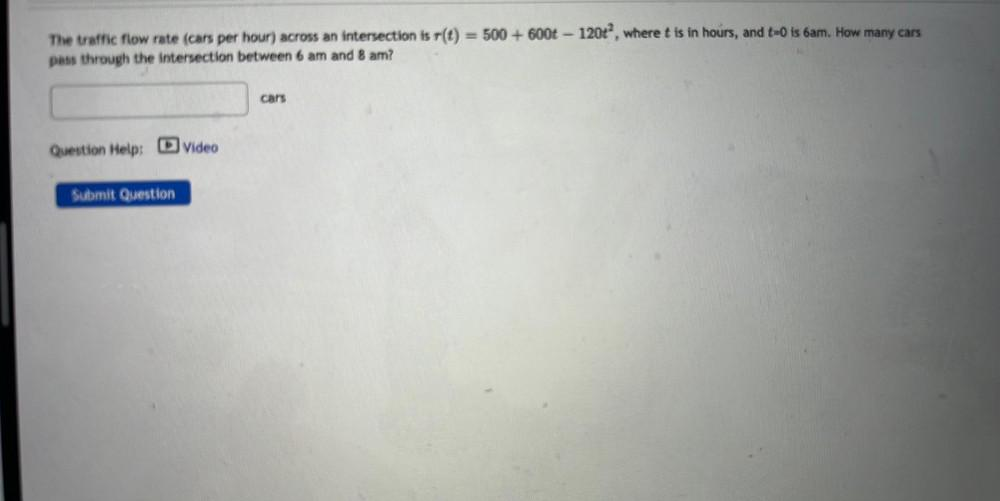Question:

# The traffic flow rate (cars per hour) across an intersection is r(t) = 500 + 6001 - 120t^2, where t is in hours, and t=0 is 6am. How many cars pass through the intersection between 6 am and 8 am?The traffic flow rate (cars per hour) across an intersection is r(t) = 500 + 6001 - 120t^2, where t is in hours, and t=0 is 6am. How many cars pass through the intersection between 6 am and 8 am?